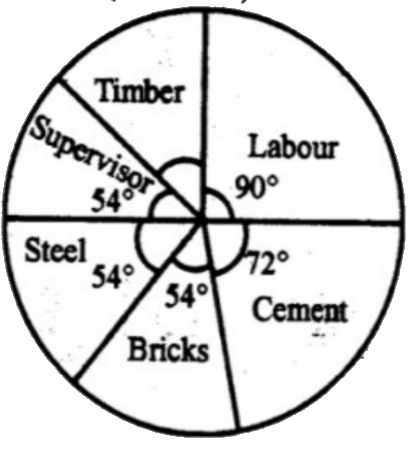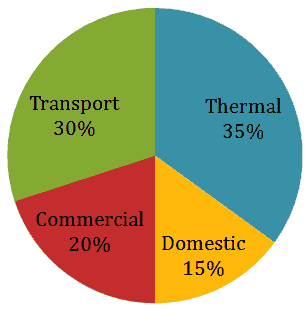@CSAT/IBPS aspirants. First solve these two DI (Data Interpretation) sets on your own, using pen and paper. Then scroll down and check answer/explanations/shortcuts.
Time: 30 mins. Correct Ans: 1 m, Wrong answer=-0.33 m.

## Data Interpretation Set#1: Pie Chart

The following pie chart cost of constructing one house. The total cost was Rs. 6 lakhs. (source FCI/SSC Nov. 2012 Paper)1. The amount spent on cement is Rs.2,00,000 Rs.1,60,000 Rs.1,20,000 Rs.1,00,000 2. The amount spent on labour exceeds the amount spent on steel by 5% of the total cost 10% of the total cost 12% of the total cost 15% of the total cost 3. The amount spent on cement, steel and supervision is what percent of the total cost of construction? 40% 45% 50% 55% 4. The amount spent on labour exceeds the amount spent on supervision by Rs.2,00,000 Rs.16,000 Rs.1,20,000 Rs.60,000 5. The amount spent on Timber is Rs.60,000 Rs.90,000 Rs.1,20,000 Rs.36,000

## DI Set#2: Pie-Chart CO2 Emission

The total annual CO2 emissions from various sectors are 5 mmt. In the Pie Chart given below, the percentage contribution to CO2 emissions from  various sectors is indicated.1. Which of the following sectors together emit 2.5 mmt of CO2 every year? Thermal and Transport Domestic and Commercial Transport and Commericial Commercial and Thermal 2. Which of the following sectors have emission difference of 1 mmt between them. Domestic and Commercial Transport and Commercial Thermal and Domestic Thermal and Transport.

3. Emission of Domestic sector is how much % of Transport and Commercial sector combined?

1. 20%
2. 15%
3. 30%
4. 35%

4. In the next year, if emission from Commercial sector decreases by 20% of its current emission, while other sectors continue to emit same amount of CO2 as earlier then What will be the new % contribution of Thermal power sector (approximatly)?

1. 31.9%
2. 39.8%
3. 42.7%
4. 36.5%

5. Which of the following is incorrectly matched?

a) Domestic=54 degrees.
b) Thermal=125 degrees.
c) commercial =72 degrees.
d) transport=108 degrees.

## DI Set #3: Bar graph on Profit-loss

Observe this graph and answer questions (source FCI/SSC Nov. 2012 Paper)1. The amount of maximum profit as seen from the diagram is 2 thousand rupees 1.5 thousand rupees 1 thousand rupees 4.5 thousand rupees 2. The amount of total loss incurred during 2005-2010 is 3 thousand rupees 2.5 thousand rupees 1.5 thousand rupees 2 thousand rupees 3. The ratio of the maximum profit earned to the minimum loss suffered is 4 : 3 3 : 4 9 : 2 2 : 9 4. If the loss is x% of the profit for the years under study, then x is 15 25 20 19

## Solutions for the DI questions

Solution DI Set#1: Pie Chart with Explainations/Shortcuts
You might be tempted to transform degrees into percentages and find absolute Rupee value of each item (timber, labour etc). But in the exam, don’t waste time by calculating everything. Only calculate the stuff that is asked.1. The amount spent on cement is

1. Rs.2,00,000
2. Rs.1,60,000
3. Rs.1,20,000
4. Rs.1,00,000

Total is Rs. 6,00,000 (=360 degrees of the circle)
Cement is 72 Degrees.
Apply the ratio principle

 Degrees Value 72 (Cement) M? 360(total) 6 lakhs

Divide left column on one side and right column on the other side.
72/360= M/6 lakhs
M=(72*6)/360=1.2 lakhs.
2. The amount spent on labour exceeds the amount spent on steel by

1. 5% of the total cost
2. 10% of the total cost
3. 12% of the total cost
4. 15% of the total cost

There is no need to find absolute values. Just observe the degrees.

 Item Degrees Labour 90 Steel (Base %) 54 Difference 36

So the difference between Labour and Steel is 36 degrees.
And total cost is (6lakh=) 360 degrees.
So the percentage= 36/360 x 100 =10% of the total cost.
3. The amount spent on cement, steel and supervision is what percent of the total cost of construction?

1. 40%
2. 45%
3. 50%
4. 55%

Again no need to find absolute values. Just observe the degrees.

 degrees Cement 72 Steel 54 Supervision 54 Total 180

He is asking, “what percent of the total cost of construction?”
So total cost (360 degrees) is the “base”
%=(180/360) x 100
=50%
4. The amount spent on labour exceeds the amount spent on supervision by

1. Rs.2,00,000
2. Rs.16,000
3. Rs.1,20,000
4. Rs.60,000

Again no need to find absolute values for both items. Just observe the degrees.
Difference between labour and supervision
=90-54
=36 degrees
Shortcut: 36 degrees =10% of a circle. (because total is 360 degrees)
So the amount spend = 10% of total (6,00,000)= one decimal leftwards=60,000.
If shortcut is not clicking your mind, no problem. Go by ratio principle

 Degrees Value 36 (difference between labor and supervision) M? 360 (total) 6 lakhs

Divide left column on one side and right column on the other side.
36/360= M/6 lakhs
M=(36*600000)/360
M=60,000
5. The amount spent on Timber is

1. Rs.60,000
2. Rs.90,000
3. Rs.1,20,000
4. Rs.36,000

Observe that degree/percentage of Timber is not given the chart.
So, first task is to find degrees of timber.
In a circle Total=360 degrees.
So Timber’s degree
=360 MINUS the degrees of remaining items
=360-(54+54+54+72+90)
=36 degrees.
Now use the concept given in previous question,
36 degrees= 10% of total cost=60,000 rupees.

## Solution: Set #2: CO2 Emission Pie Chart1. Which of the following sectors together emit 2.5 mmt of CO2 every year?

1. Thermal and Transport
2. Domestic and Commercial
3. Transport and Commercial
4. Commercial and Thermal

Total emission is 5. And 2.5mmt is 50% (half) of 5 (total emission)

From the given circle, transport + commercial, represent half circle (50%) Similarly Thermal+Domestic is also 50%. But it is not given in options. So answer is (C): Transport + Commercial.

2. Which of the following sectors have emission difference of 1 mmt between them.

1. Domestic and Commercial
2. Transport and Commercial
3. Thermal and Domestic
4. Thermal and Transport.
• Total is 5mmt (100%) So 1mmt=1 x 100/5=20%.
• Find out which two sectors have % difference of 20%?
• Thermal minus Domestic=35-15=20%. So (C) is the answer.

3. Emission of Domestic sector is how much % of Transport and Commercial sector combined?

1. 20%
2. 15%
3. 30%
4. 35%

Observe the chart

Required %

=(15/50) x 100

4. In the next year, if emission from Commercial sector decreases by 20% while other sectors continue to emit same amount of CO2 as earlier then What will be the new % contribution of Thermal power sector?

Right now Commercial sector emits 20% of 5mmt=1/5 x 5=1mm

Next year 20% decrease=20% of 1mm=0.2mm will be less emitted.

But other sectors remains the same.

So total emission next year=5 minus 0.2=4.8mm.

And Share of thermal power

=(35% of 5)/4.8

=0.35*5/4.8

=36.5% (if you had trouble in long-division, use the speed maths %approximation trick CLICK ME)

5. Which of the following is incorrectly matched?

a) Domestic=54 degrees.
b) Thermal=125 degrees.
c) commercial =72 degrees.
d) transport=108 degrees.

100%=360 degrees So 1%=3.6 degree.

Multiply every sector’s % with 3.6 and you’ll get corresponding degree.

Ans (B) because correct degree of Thermal should be 35 x 3.6=126 degrees.

## Solution :Set #3 Bar Chart (With explaination)

Be careful about the ‘unit’, Thousand/lakhs.1. The amount of maximum profit as seen from the diagram is

1. 2 thousand rupees
2. 1.5 thousand rupees
3. 1 thousand rupees
4. 4.5 thousand rupees

The bar of 2008 is longest among all. So 4.5 Ans (D)
2. The amount of total loss incurred during 2005-2010 is

1. 3 thousand rupees
2. 2.5 thousand rupees
3. 1.5 thousand rupees
4. 2 thousand rupees

Loss occurs during 2006 and 2009.

 Year Loss 2006 1.5 2009 1.0 Total 2.5 answer (B)

3. The ratio of the maximum profit earned to the minimum loss suffered is

1. 4 : 3
2. 3 : 4
3. 9 : 2
4. 2 : 9
 Max profit (2008) 4.5 Min Loss (2009) 1

Ratio=4.5/1=45/10=9/2
4. If the loss is x% of the profit for the years under study, then x is

1. 15
2. 25
3. 20
4. 19

We’ve to take the total of profits and losses made in all years so,

 Profit Loss 2005 2.5 0 06 0 1.5 07 3.5 0 08 4.5 0 09 0 1 10 2 0 Total 12.5 2.5

Profit: Loss
=2.5/12.5
=1/5
=20% (remember the fraction to percentage table: Click ME.)